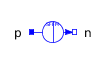Relative Rotation - MapleSim Help

Relative Rotation

Measures the relative rotational motion between two framesDescription A relative rotation sensor measures the rotation matrix, angular velocity, and angular acceleration of frame_b with respect to frame_a.  The sensor generates three vector outputs (a length 9 vector (column-major order) and two 3-vectors) containing these measurements, expressed in the frame specified by the Resolved Frame parameter. (Applies to the angular velocity and acceleration only. This parameter is ignored for the rotation matrix output.)Connections

 Name Description ${\mathrm{frame}}_{a}$ Sensor frame ${\mathrm{frame}}_{b}$ Sensor frame $\mathrm{RMOutputR}$ Real signal of dimension 9 (column-major order) that represents the rotation matrix between the two frames. $\mathrm{RMOutputW}$ Real signal of dimension 3 that specifies the angular velocity of frame_b with respect to frame_a.  The measured quantity is expressed in the frame specified by the parameter Resolved Frame. $\mathrm{RMOutputAA}$ Real signal of dimension 3 that specifies the angular acceleration frame_b with respect to frame_a.  The measured quantity is expressed in the frame specified by the parameter Resolved Frame.Parameters

 Symbol Default Units Description Modelica ID Resolved Frame Inertial Frame in which the output signals are expressed. FrameSee Also